# Question 15 Given that f(x)2x + 3, and g(x)=2x2 – 1. Determine (gof)(x), expand, and simplify....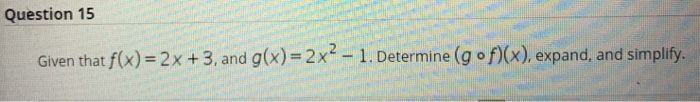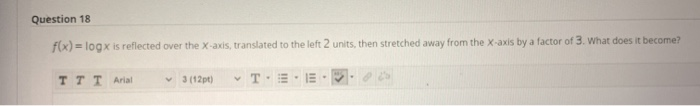Question 15 Given that f(x)2x + 3, and g(x)=2x2 – 1. Determine (gof)(x), expand, and simplify.
Question 18 f(x) = log x is reflected over the x-axis, translated to the left 2 units, then stretched away from the X-axis by a factor of 3. What does it become? TTT Ariat 3 (12pt)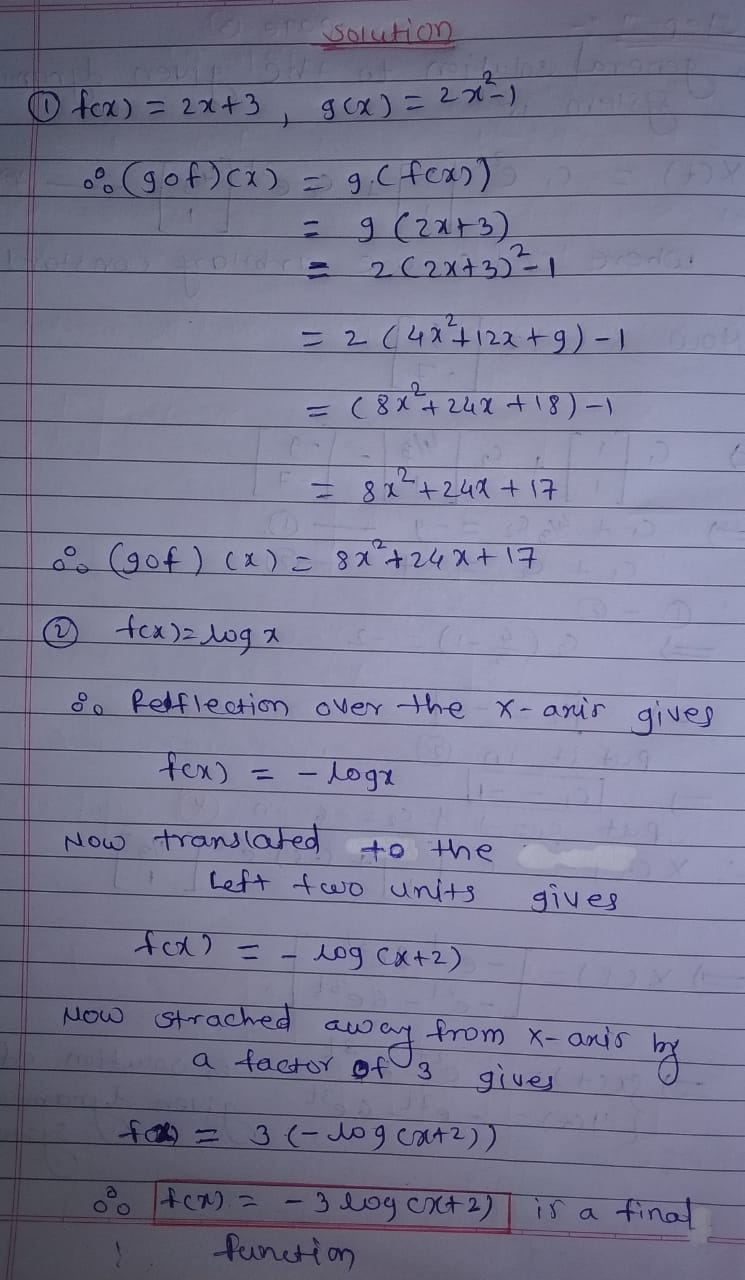##### Add Answer of: Question 15 Given that f(x)2x + 3, and g(x)=2x2 – 1. Determine (gof)(x), expand, and simplify....
Similar Homework Help Questions
• ### Question 16 Given that f(x)= - 3x2 - 6x + 2. Determine the vertex. Question 17...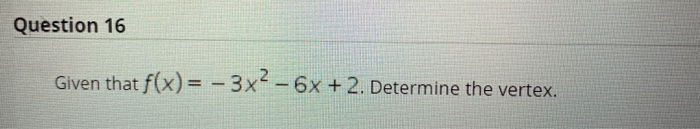Question 16 Given that f(x)= - 3x2 - 6x + 2. Determine the vertex. Question 17 x²+2 Given that log3(x2 + 2) - log3(x + 4) = 1. What numerical value is equal to? ( You are not asked to solve the equation.) X + 4 Question 18 f(x) = log x is reflected over the x-axis, translated to the left 2 units, then stretched away from the X-axis by a factor of 3. What does it become?

• ### Find (fog)(x) and (gof)(x) and the domain of each f(x) = x²-6, g(x) = 2x -...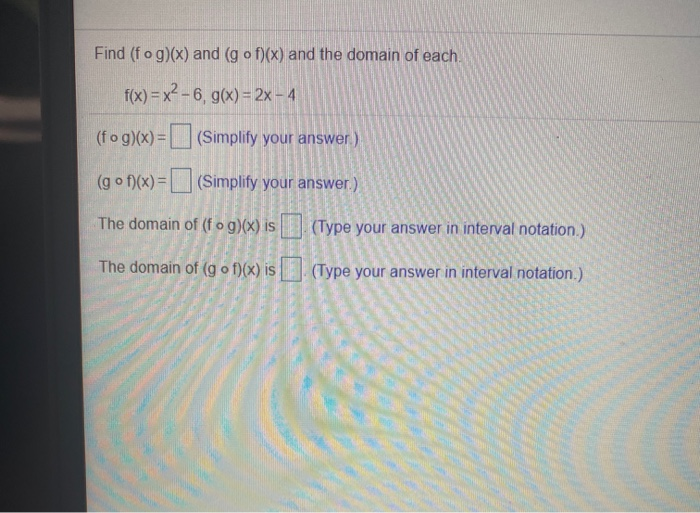Find (fog)(x) and (gof)(x) and the domain of each f(x) = x²-6, g(x) = 2x - 4 (fog)(x) = (Simplify your answer) (gof)(x) = (Simplify your answer.) The domain of (fog)(x) is (Type your answer in interval notation.) The domain of (gof)(x) is (Type your answer in interval notation.)

• ### Question 12 X-2 Solve X +3 SO. Express then solution in interval notation. Question 13 x2...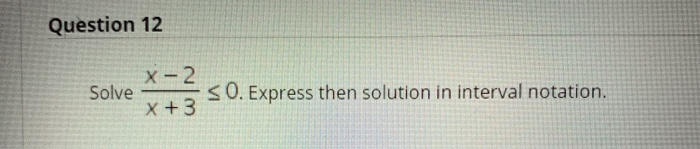Question 12 X-2 Solve X +3 SO. Express then solution in interval notation. Question 13 x2 - 4x +3 Determine the domain of f(x)= in interval notation. X - 1 Question 14 Given that f(x) = - 4x + 3 is a one-to-one function. Determine f-1(x). Question 15 Given that f(x) = 2x + 3, and g(x)=2x2 – 1. Determine (gof)(x), expand, and simplify.

• ### Given the function f(x) = -2x? +12x - 5, describe the transformations compared to the parent...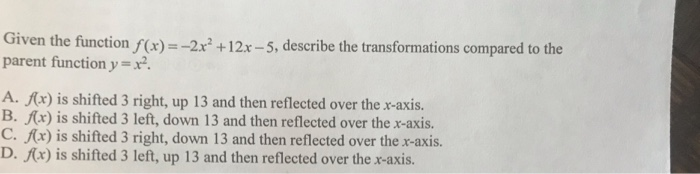Given the function f(x) = -2x? +12x - 5, describe the transformations compared to the parent function y = x2. A. f(x) is shifted 3 right, up 13 and then reflected over the x-axis. B. f(x) is shifted 3 left, down 13 and then reflected over the x-axis. C. f(x) is shifted 3 right, down 13 and then reflected over the x-axis. D. f(x) is shifted 3 left, up 13 and then reflected over the x-axis.

• ### please answer ❤️ For f(x) = 2x and g(x)= x + 2, find the following functions....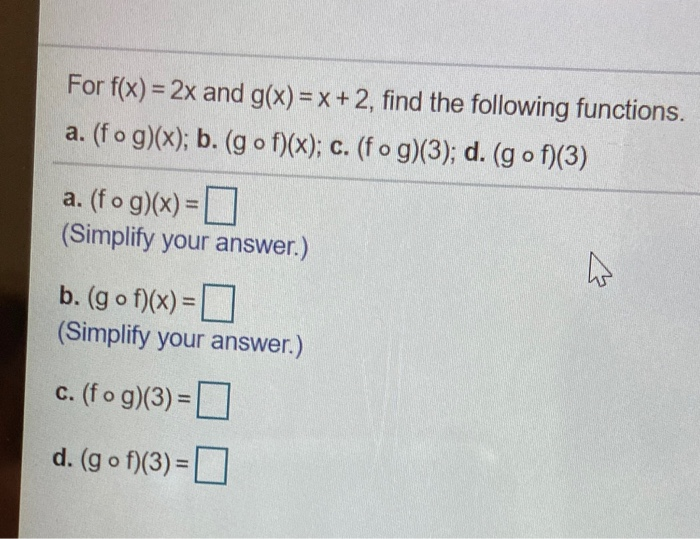please answer ❤️ For f(x) = 2x and g(x)= x + 2, find the following functions. a. (fog)(x); b. (gof)(x); c. (fog)(3); d. (gof)(3) a. (fog)(x) = (Simplify your answer.) b. (gof)(x) = 0 (Simplify your answer.) C. (fog)(3) = d. (gof)(3)=0

1Marks: --/10Identify the function for the graph described:the graph of f(x) = x2 is horizontally compressed by a factor of ?.Choose one answer.a. g(x) = 3x2b. g(x) = (?x)2c. g(x) = (3x)2d. g(x) = ?x2Question 2Marks: --/10Identify the transformation from the parent function f(x) = x2 to g(x) = x2 + 3.Choose one answer.a. a vertical translation of 3 units upb. a horizontal translation of 3 units leftc. a vertical translation of 3 units downd. a horizontal translation of 3...

1Marks: --/10Identify the transformation from the parent function f(x) = x2 to g(x) = -¼x2.Choose one answer.a. vertical stretch by a factor of ¼ and a reflection across the x-axisb. vertical compression by a factor of 4 and a reflection across the x-axisc. vertical compression by a factor of ¼ and a reflection across the x-axisd. vertical stretch by a factor of 4 and a reflection across the x-axisQuestion 2Marks: --/10Identify the transformation from the parent function f(x) = x2...

• ### How would I write this as a function of y=x^3 Reflected in the y axis, horizontally stretched by a factor of 7, vertically translated 19 units down

How would I write this as a function of y=x^3Reflected in the y axis, horizontally stretched by a factor of 7, vertically translated 19 units down.Would this be correct? g(x)= (-1/7(x-1))^3-19

• ### Question 15 1 pt 1 Details -3.2 - 7 Given the function f(x) = - 2x2...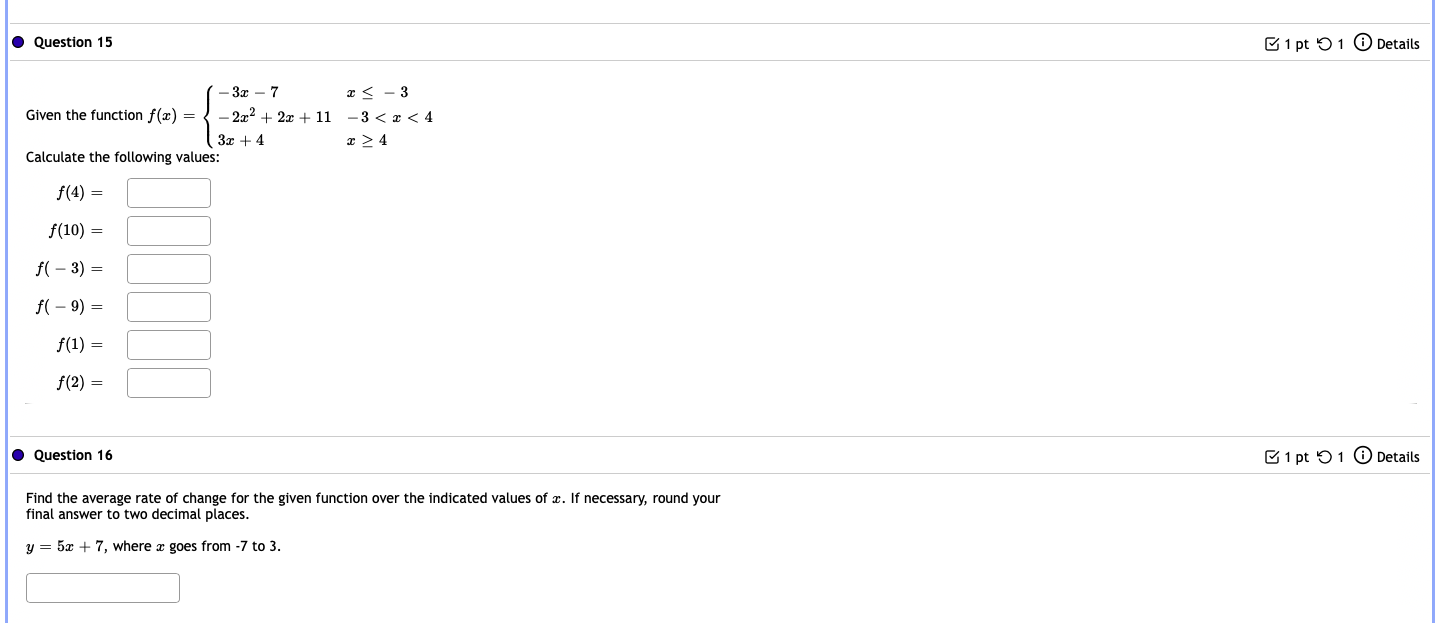Question 15 1 pt 1 Details -3.2 - 7 Given the function f(x) = - 2x2 + 2x + 11 32 +4 Calculate the following values: <-3 -3<<4 f(4) = f(10) = f(-3) = fl - 9) = f(1) = f(2) = Question 16 1 pt 1 Details Find the average rate of change for the given function over the indicated values of x. If necessary, round your final answer to two decimal places. y = 5x + 7, where...